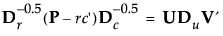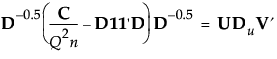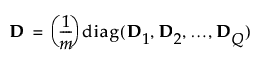Publication date: 08/13/2020

## Details Report

When a simple Correspondence Analysis is performed, the report lists the singular values of the following equation:where:

P is the matrix of counts divided by the total frequency

r and c are the row and column sums of P

the D matrices are diagonal matrices of the values of r and c

When Multiple Correspondence Analysis is performed, the singular value decomposition extends to the following equation:where:C is the Burt table.

Q is the number of categorical variables

n is the number of observations

1 is a column vector of ones